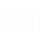## Data mining normalizationIf you have ever dealt with databases, you are probably familiar with the term «data mining normalization». There is the view that data normalization is a luxury that only academics can afford. Ordinary users have no time for it. Nevertheless, the awareness of the principles of data mining normalization and the adoption of them in work with databases enables to enhance the quality of data processing with little or no difficulty.

In this article, we will look at the essence of data mining normalization and most often encountered approaches to this process.## What is data mining normalization?

The data normalization (also referred to as data pre-processing) is a basic element of data mining. It means transforming the data, namely converting the source data in to another format that allows processing data effectively. The main purpose of data normalization is to minimize or even exclude duplicated data. This is a very essential and important issue because it is increasingly problematic to keep in data in relational databases, which store identical data in more than one place.

The use of data mining normalization has a number of advantages:

• the application of data mining algorithms becomes easier
• the data mining algorithms get more effective and efficient
• the data is converted in to the format that everyone can get their heads around
• the data can be extracted from databases faster
• it is possible to analyze the data in a specific manner

As you can see applying data mining normalization is reasonable and allows achieving certain results. There are some data mining normalization techniques that are widely used for the data transformation and which will be discussed below.

## Min-Max normalization

The first technique we will cover is min-max normalization. It is the linear transformation of the original unstructured data. It scales the data from 0 to 1. It is calculated by the following formula:where is the current value of feature F.

Let us consider one example to make the calculation method clear. Assume that the minimum and maximum values for the feature F are \$50,000 and \$100,000 correspondingly. It needs to range F from 0 to 1. In accordance with min-max normalization, v = \$80,000 is transformed to:As you can see this technique enables to interpret the data easily. There are no large numbers, only concise data that do not require further transformation and can be used in decision-making process immediately.

## Z-score normalization

The next technique is z-score normalization. It is also called zero-mean normalization. The essence of this technique is the data transformation by the values conversation to a common scale where an average number equals zero and a standard deviation is one. A value is normalized to ′ under the formula:Here is the mean and is the standard deviation of feature F.

Here is an example of the calculation of a value.

On the supposition that the mean of feature is \$65,000 and its standard deviation is \$ 18,000. Applying the z-score normalization we get the following mean of the value equals to \$85,800:Therefore, z-score normalization is an approach to the data processing that takes into account outliers; however, it does not provide data normalization with an identical scale.

## Data normalization by decimal scaling

And now we finally will move on to the decimal scaling normalization technique. It involves the data transformation by dragging the decimal points of values of feature F. The movement of decimals is very dependent on the absolute value of the maximum. A value of feature F is transformed to by calculating:In this formula, j is the lowest integer while Max (|) < 1.

For instance, values for feature F scale from 850 to 825. Suppose j equals to three. In this case, the maximum absolute value of feature F equals 850. Applying the normalization with decimal scaling, we need to divide all values by 1,000. Therefore, we get 850 normalized to 0,850 as well as 825 transformed to 0,825.

This technique entails the transformation of the decimal points of the values according to the absolute value of the maximum. It follows that the means of the normalized data will always be between 0 and 1.

## Conclusion

To sum up, the data normalization is an approach of data organization in multiple related databases. It provides the transformation of tables in order to avoid data redundancy and undesirable properties such as anomalies of insertion, update, and deletion. Data mining normalization is a multi-stage process that transforms data into the table deleting repeated data from the relational databases. It is very important because if the dataset is great that includes many fine features but it is not normalized one of the features can prevail over other ones. Data mining normalization solves this problem.Write US